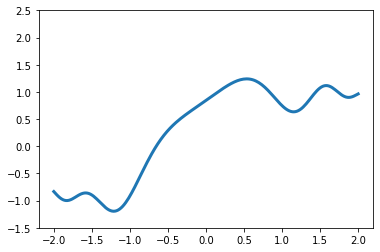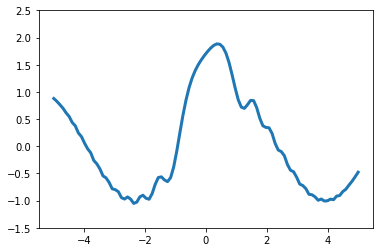Related Articles
MoviePy – Creating Animation Using Matplotlib
• Last Updated : 18 Aug, 2020

In this article we will see how we create animations in MoviePy using matplotlib. MoviePy is a Python module for video editing, which can be used for basic operations on videos and GIF’s. Video is formed by the frames, combination of frames creates a video each frame is an individual image. Matplotlib is a plotting library for the Python programming language and its numerical mathematics extension NumPy. It provides an object-oriented API for embedding plots into applications using general-purpose GUI toolkits like Tkinter, wxPython, Qt, or GTK+.

In order to do this we have to do the following
1. Import matplotlib modules
2. Import moviepy modules
3. Create a numpy array
4. Create a subplot using matplotlib
5. Create a Video clip file by calling the make_frame method
6. Inside the the make frame method
7. Clear the plot and create a new plot using trigonometry methods according to the frame time
8. Return the plot after converting it into numpy image.

Below is the implementation

 `# importing matplot lib``import` `matplotlib.pyplot as plt``import` `numpy as np`` ` `# importig movie py libraries``from` `moviepy.editor ``import` `VideoClip``from` `moviepy.video.io.bindings ``import` `mplfig_to_npimage`` ` `# numpy array``x ``=` `np.linspace(``-``2``, ``2``, ``200``)`` ` `# duration of the video``duration ``=` `2`` ` `# matplot subplot``fig, ax ``=` `plt.subplots()`` ` `# method to get frames``def` `make_frame(t):``     ` `    ``# clear``    ``ax.clear()``     ` `    ``# plotting line``    ``ax.plot(x, np.sinc(x``*``*``2``) ``+` `np.sin(x ``+` `2` `*` `np.pi ``/` `duration ``*` `t), lw ``=` `3``)``    ``ax.set_ylim(``-``1.5``, ``2.5``)``     ` `    ``# returning mumpy image``    ``return` `mplfig_to_npimage(fig)`` ` `# creating animation``animation ``=` `VideoClip(make_frame, duration ``=` `duration)`` ` `# displaying animation with auto paly and looping``animation.ipython_display(fps ``=` `20``, loop ``=` `True``, autoplay ``=` `True``)`

Output :

```Moviepy - Building video __temp__.mp4.
Moviepy - Writing video __temp__.mp4

Moviepy - Done !
Moviepy - video ready __temp__.mp4
```Another example

 `# importing matplot lib``import` `matplotlib.pyplot as plt``import` `numpy as np`` ` `# importig movie py libraries``from` `moviepy.editor ``import` `VideoClip``from` `moviepy.video.io.bindings ``import` `mplfig_to_npimage`` ` `# numpy array``x ``=` `np.linspace(``-``5``, ``5``, ``100``)`` ` `# duration of the video``duration ``=` `2`` ` `# matplot subplot``fig, ax ``=` `plt.subplots()`` ` `# method to get frames``def` `make_frame(t):``     ` `    ``# clear``    ``ax.clear()``     ` `    ``# plotting line``    ``ax.plot(x, np.sinc(x``*``*``2``) ``+` `np.cos(x ``+` `10` `*` `np.pi ``/` `duration ``*` `t), lw ``=` `3``)``    ``ax.set_ylim(``-``1.5``, ``2.5``)``     ` `    ``# returning mumpy image``    ``return` `mplfig_to_npimage(fig)`` ` `# creating animation``animation ``=` `VideoClip(make_frame, duration ``=` `duration)`` ` `# displaying animation with auto paly and looping``animation.ipython_display(fps ``=` `20``, loop ``=` `True``, autoplay ``=` `True``)`

Output :

```Moviepy - Building video __temp__.mp4.
Moviepy - Writing video __temp__.mp4

Moviepy - Done !
Moviepy - video ready __temp__.mp4
```Attention geek! Strengthen your foundations with the Python Programming Foundation Course and learn the basics.

To begin with, your interview preparations Enhance your Data Structures concepts with the Python DS Course.

My Personal Notes arrow_drop_up